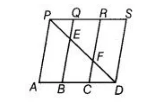# In the figure, PSDA is a parallelogram.`
Question:

In the figure, PSDA is a parallelogram. Points Q and R are taken on PS such that PQ = QR= RS and PA || QB || RC. Prove that ar (PQE) =ar (CFD).Thinking Process

1. Firstly, use the formula, area of parallelogram = Base x Altitude
2. Further, prove that A PQE = AD CF, by ASA congruent rule.
3. At the end use the property that congruent figures have same area.

Solution:

Given In a parallelogram PSDA, points 0 and R are on PS such that

PQ = QR = RS and PA || QB || RC.

To prove ar (PQE) = ar (CFD)

Proof In parallelogram PABQ,

and PA||QB [given]

So, PABQ is a parallelogram.

PQ = AB …(i)

Similarly, QBCR is also a parallelogram.

QR = BC …(ii)

and RCDS is a parallelogram.

RS =CD …(iii)

Now, PQ=QR = RS …(iv)

From Eqs. (i)(ii) (iii) and (iv),

PQ || AB [∴ in parallelogram PSDA, PS || AD]

In ΔPQE and ΔDCF, ∠QPE = ∠FDC

[since, PS || AD and PD is transversal, then alternate interior angles are equal] PQ=CD [from Eq. (v)]

and ∠PQE = ∠FCD

[∴ ∠PQE = ∠PRC corresponding angles and ∠PRC = ∠FCD alternate interior angles]

ΔPQE = ΔDCF [by ASA congruence rule]

∴ ar (ΔPQE) = ar (ΔCFD) [since,congruent figures have equal area]

Hence proved.